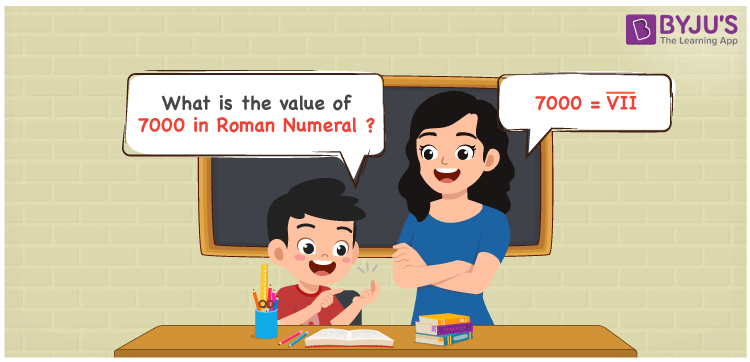# 7000 in Roman Numerals

7000 in Roman Numerals is equal to V̅I̅I̅. The procedure of expressing the numbers using the Roman alphabet is known as Roman Numerals. Roman Numerals 1 to 1000 can be downloaded anytime free of cost to learn the Roman Numerals for the numbers 1 to 1000 accurately. Let us learn in detail how to denote the number 7000 in Roman Numerals in this article.

 Number Roman Numeral 7000 V̅I̅I̅

## How to Write 7000 in Roman Numerals?In order to represent 7000 in Roman Numerals, we use vinculum over the Roman Numeral ‘VII’. Vinculum is used to represent the multiples of 1000’s in Roman Numerals. Hence, adding a vinculum over 7 is equal to 7 x 1000 = 7000. Thus, the value of 7000 in Roman Numerals is V̅I̅I̅.

## Video Lesson on Roman Numerals## Frequently Asked Questions on 7000 in Roman Numerals

Q1

### What is 7000 in Roman Numerals?

7000 in Roman Numerals is V̅I̅I̅.
Q2

### Express the value of 1000 + 6000 in Roman Numerals.

The value of 1000 + 6000 is 7000. Hence 7000 in Roman Numerals is V̅I̅I̅. Thus, the value of 1000 + 6000 in Roman Numerals is V̅I̅I̅.
Q3

### What should be added to 6000 to get 7000? Express the answer in Roman Numerals.

7000 – 6000 = 1000. So, 1000 should be added to 6000 to get the number 7000. Therefore 1000 in Roman Numerals is M.